## 插入节点#

1. 找到要插入的位置，这个位置就是当前这棵完全二叉树，最后一个位置 (不太好理解)。如果用代码流程来描述的话，就是按层遍历当前的树，如果遇到一个节点，左子树为空，或者又子树为空，或者左右子树都为空，那么，这个点就是要插入节点的父节点，而要插入的节点，将作为这个节点的左子树或右子树。看下面的图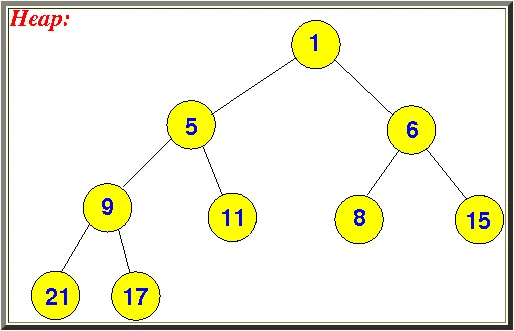1. 找到了要插入节点的父节点，还要判断这个父节点是左子树为空，还是右子树为空，还是都为空，如果左子树为空，则新点作为左子树，如果左子树不为空，则新点作为右子树。插入后的图如下(假设要插入的数值为3)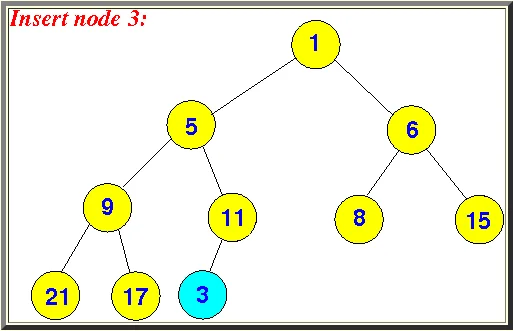1. 节点插入后，当前的树并不满足二叉堆的规则，所以还需要将新插入的点向上浮动。如果当前节点值小于父节点的值，则交换当前节点和父节点的值。然后再次判断，直到当前值不再小于父节点值，或者父节点值为空了，则位置调整完毕，整个插入流程也就结束了。看下面的流程图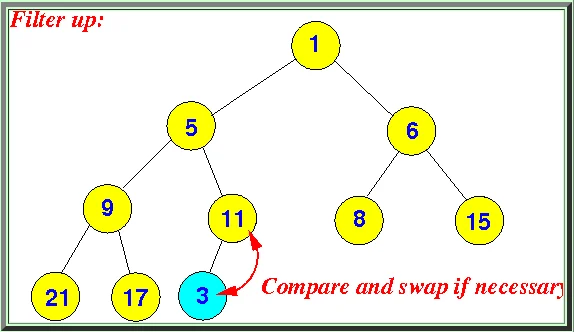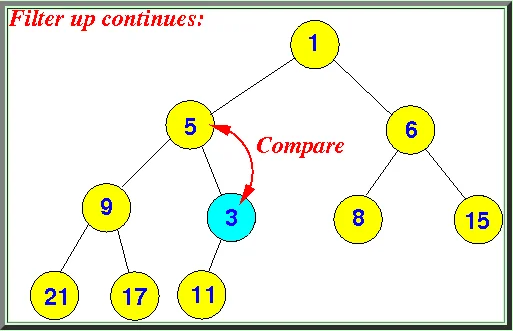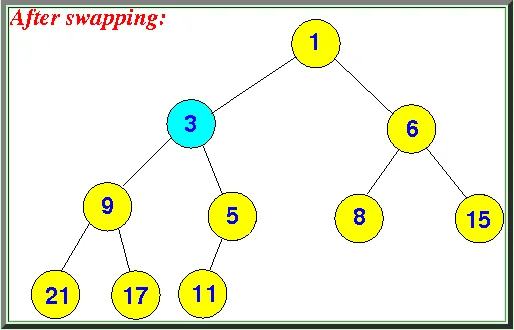// 定义节点的结构
public class Node {
public int data;
public Node parent = null;
public Node left = null;
public Node right = null;

public Node(int data){
this.data = data;
}
}

// 定义二叉堆操作类
using System;

public class BinaryHeap {
public Node root;

public void Insert(int data){
Node lastParentNode = TreeAlgo.FindParentOfFartestLeftLocation(root);
if(lastParentNode == null){
Console.WriteLine("Insert Root Node " + data);
root = new Node(data);
}else{
Node newNode = new Node(data);
if(lastParentNode.left == null){ // 如果左子树为空，则插入到左子树
lastParentNode.left = newNode;
} else if(lastParentNode.right == null){ // 如果右子树为空，则插入到右子树
lastParentNode.right = newNode;
}else{
Console.WriteLine("父节点错误，父节点左右子树都不为空，无法插入!");
return;
}

newNode.parent = lastParentNode;

Node opt = newNode;
// 以最小堆的方式插入节点，即父节点小于等于子节点
// 如果是以最大堆的方式插入，则父节点要大于等于子节点 只要将 <= 改为 >= 即可
while(opt != null && opt.parent != null && opt.data <= opt.parent.data){
int tmp = opt.data;
opt.data = opt.parent.data;
opt.parent.data = tmp;
opt = opt.parent;
}
}
}
}

// 运行刚才写的代码
using System;
using System.Collections.Generic;

class Program
{
static void Main(string[] args)
{
BinaryHeap bh = new BinaryHeap();
bh.Insert(1);
bh.Insert(9);
bh.Insert(22);
bh.Insert(17);
bh.Insert(11);
bh.Insert(33);
bh.Insert(27);
bh.Insert(21);
bh.Insert(19);
TreeAlgo.LevelOrderTraversal(bh.root);
}
}

// TreeAlgo 帮助类代码
using System;
using System.Collections.Generic;
public static class TreeAlgo {
// 层序遍历树，打印出的内容，括号里面是父节点的值
public static void LevelOrderTraversal(Node root){
if(root == null){
return;
}

Queue<Node> q = new Queue<Node>();
q.Enqueue(root);

while(q.Count > 0){
Node currNode = q.Dequeue();
string msg =string.Format("{0}({1}) ", currNode.data, currNode.parent != null?currNode.parent.data.ToString():"null");
Console.WriteLine(msg);
if(currNode.left != null){
q.Enqueue(currNode.left);
}

if(currNode.right != null){
q.Enqueue(currNode.right);
}
}
}

// 按层遍历，获取第 index 个节点
public static Node GetIndexOfNodeWithLevelOrder(Node root, int index){
if(root == null){
return null;
}

Queue<Node> q = new Queue<Node>();
q.Enqueue(root);
int iIndex = 0;
Node targetNode = null;
while(q.Count > 0){
Node currNode = q.Dequeue();
if(iIndex == index){
targetNode = currNode;
break;
}

if(currNode.left != null){
q.Enqueue(currNode.left);
}

if(currNode.right != null){
q.Enqueue(currNode.right);
}

iIndex += 1;
}

return targetNode;
}

// 获取按层遍历时，最后一层的最后一个节点
public static Node GetLastNodeOfLevelOrder(Node root){
if(root == null){
return null;
}

Queue<Node> q = new Queue<Node>();
q.Enqueue(root);
Node lastNode = null;
while(q.Count > 0){
lastNode = q.Dequeue();
if(lastNode.left != null){
q.Enqueue(lastNode.left);
}

if(lastNode.right != null){
q.Enqueue(lastNode.right);
}
}

return lastNode;
}

// 按层序遍历，只要任何一个节点左子对为空，或右子树为空，则为要找的点（前提是这棵树必须为完全二叉树）
public static Node FindParentOfFartestLeftLocation(Node root){
if(root == null){
return null;
}

Queue<Node> q = new Queue<Node>();
q.Enqueue(root);
Node targetNode = null;

while(q.Count > 0){
targetNode = q.Dequeue();
if(targetNode.left == null || targetNode.right == null){
break;
}

q.Enqueue(targetNode.left);
q.Enqueue(targetNode.right);
}

return targetNode;
}
}


## 删除节点#

1. 找到按层序遍历的最后一个节点，然后用最后一个节点的值，替换要删除节点的值。然后将最后一个节点值删除掉。看下面的图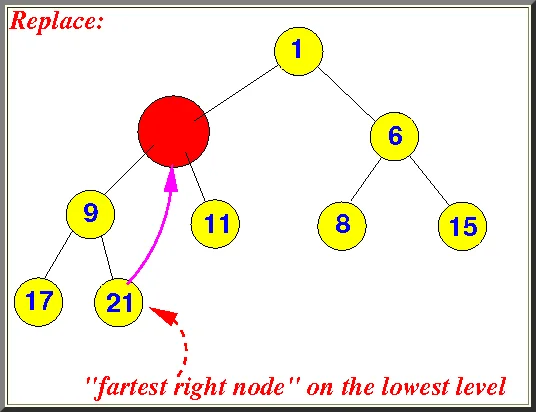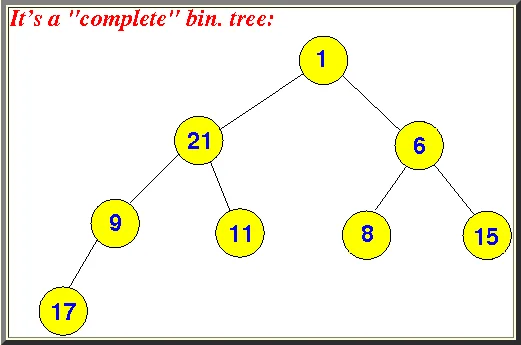1. 浮动节点，将树调整为正确的二叉堆。删除节点时，节点的浮动有可能需要往下浮动，也有可能需要往上浮动。我们当前所讲的，都是按最小堆来操作的。如果 21 节点比父节点小，则要往上浮动。如果 21 节点比父节点大，则要往下浮动。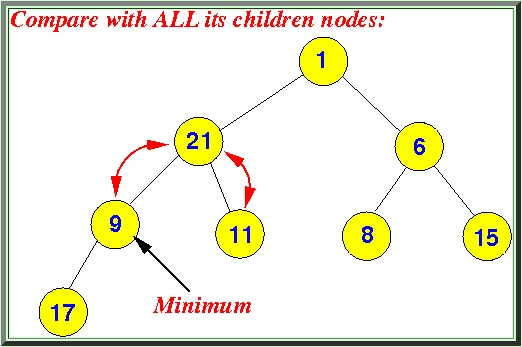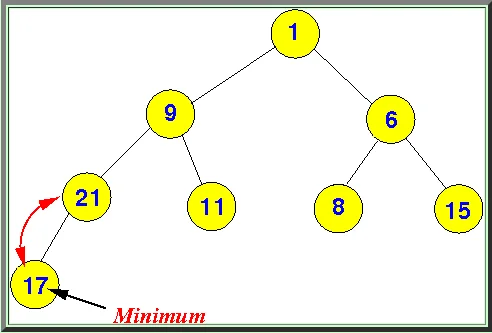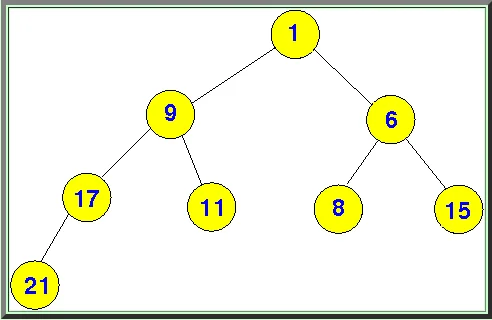using System;

public class BinaryHeap {
public Node root;

public void Insert(int data){
Node lastParentNode = TreeAlgo.FindParentOfFartestLeftLocation(root);
if(lastParentNode == null){
Console.WriteLine("Insert Root Node " + data);
root = new Node(data);
}else{
Node newNode = new Node(data);
if(lastParentNode.left == null){ // 如果左子树为空，则插入到左子树
lastParentNode.left = newNode;
} else if(lastParentNode.right == null){ // 如果右子树为空，则插入到右子树
lastParentNode.right = newNode;
}else{
Console.WriteLine("父节点错误，父节点左右子树都不为空，无法插入!");
return;
}

newNode.parent = lastParentNode;

Node opt = newNode;
// 以最小堆的方式插入节点，即父节点小于等于子节点
// 如果是以最大堆的方式插入，则父节点要大于等于子节点 只要将 <= 改为 >= 即可
while(opt != null && opt.parent != null && opt.data <= opt.parent.data){
int tmp = opt.data;
opt.data = opt.parent.data;
opt.parent.data = tmp;
opt = opt.parent;
}
}
}

public void Delete(int index){
// 1. 找到要删除的节点
Node optNode = TreeAlgo.GetIndexOfNodeWithLevelOrder(root, index);

// 2. 按层序遍历，找到最后一层最后一个节点
Node lastNode = TreeAlgo.GetLastNodeOfLevelOrder(root);

// 3. 使用最后一个节点的数据替换掉要删除节点的数据
optNode.data = lastNode.data;

// 4. 把最后一层最后一个节点删除掉
if(lastNode.parent.left == lastNode){
lastNode.parent.left = null;
}else if(lastNode.parent.right == lastNode){
lastNode.parent.right = null;
}

// 5. 要删除的节点数据已经被替换，现在需要根据数据浮动到正确的位置
// 首先要判断是往下浮动还是往上浮动，因为我们是按最小堆操作的，
// 所以要先判断当前节点是否比父节点 "小"，如果是，则要往上浮动，反之往下浮动
if(optNode.parent != null && optNode.data < optNode.parent.data){
while(optNode.parent != null && optNode.data < optNode.parent.data){
int tmp = optNode.data;
optNode.data = optNode.parent.data;
optNode.parent.data = tmp;

optNode = optNode.parent;
}
}
else{
// 尝试往下浮动
while(optNode.left != null || optNode.right != null){
Node compareNode = null;
if(optNode.left != null && optNode.right != null){
if(optNode.left.data < optNode.right.data){
compareNode = optNode.left;
}else{
compareNode = optNode.right;
}
}
else{
if(optNode.left != null){
compareNode = optNode.left;
}else if(optNode.right != null){
compareNode = optNode.right;
}
}

// 往下浮动，交换数据
if(compareNode != null && optNode.data > compareNode.data){
int temp = optNode.data;
optNode.data = compareNode.data;
compareNode.data = temp;
}

optNode = compareNode;
}
}

}
}


using System;
using System.Collections.Generic;

class Program
{
static void Main(string[] args)
{
BinaryHeap bh = new BinaryHeap();
bh.Insert(1);
bh.Insert(5);
bh.Insert(6);
bh.Insert(9);
bh.Insert(11);
bh.Insert(8);
bh.Insert(15);
bh.Insert(17);
bh.Insert(21);

TreeAlgo.LevelOrderTraversal(bh.root);

bh.Delete(1);

Console.WriteLine("------ Delete 1");
TreeAlgo.LevelOrderTraversal(bh.root);
}
}